### 5.12  Module Int64

64-bit integers.

This module provides operations on the type `int64` of signed 64-bit integers. Unlike the built-in `int` type, the type `int64` is guaranteed to be exactly 64-bit wide on all platforms. All arithmetic operations over `int64` are taken modulo 264

Performance notice: values of type `int64` occupy more memory space than values of type `int`, and arithmetic operations on `int64` are generally slower than those on `int`. Use `int64` only when the application requires exact 64-bit arithmetic.

val zero : 'a int64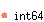The 64-bit integer 0.

val one : 'a int64The 64-bit integer 1.

val minus_one : 'a int64The 64-bit integer -1.

val neg : 'a int64 -> 'a int64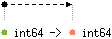Unary negation.

val add : 'a int64 -> 'a int64 -> 'a int64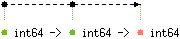val sub : 'a int64 -> 'a int64 -> 'a int64Subtraction.

val mul : 'a int64 -> 'a int64 -> 'a int64Multiplication.

val div : 'a int32 -> 'b int32 -{'c | Division_by_zero: 'c |}-> 'a int32
with 'b < 'a, 'cInteger division. Raise `Division_by_zero` if the second argument is zero. This division rounds the real quotient of its arguments towards zero, as specified for `Pervasives.(/)`.

val rem : 'a int32 -> 'b int32 -{'c | Division_by_zero: 'c |}-> 'a int32
with 'b < 'a, 'cInteger remainder.

val succ : 'a int64 -> 'a int64Successor. `Int64.succ x` is `Int64.add x Int64.one`.

val pred : 'a int64 -> 'a int64Predecessor. `Int64.pred x` is `Int64.sub x Int64.one`.

val abs : 'a int64 -> 'a int64Return the absolute value of its argument.

val max_int : 'a int64The greatest representable 64-bit integer, 263 - 1.

val min_int : 'a int64The smallest representable 64-bit integer, -263.

val logand : 'a int64 -> 'a int64 -> 'a int64Bitwise logical and.

val logor : 'a int64 -> 'a int64 -> 'a int64Bitwise logical or.

val logxor : 'a int64 -> 'a int64 -> 'a int64Bitwise logical exclusive or.

val lognot : 'a int64 -> 'a int64Bitwise logical negation

val shift_left : 'a int64 -> 'a int -> 'a int64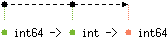`Int64.shift_left x y` shifts `x` to the left by `y` bits. The result is unspecified if `y < 0` or `y >= 64`.

val shift_right : 'a int64 -> 'a int -> 'a int64`Int64.shift_right x y` shifts `x` to the right by `y` bits. This is an arithmetic shift: the sign bit of `x` is replicated and inserted in the vacated bits. The result is unspecified if `y < 0` or `y >= 64`.

val shift_right_logical : 'a int64 -> 'a int -> 'a int64`Int64.shift_right_logical x y` shifts `x` to the right by `y` bits. This is a logical shift: zeroes are inserted in the vacated bits regardless of the sign of `x`. The result is unspecified if `y < 0` or `y >= 64`.

val of_int : 'a int -> 'a int64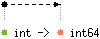Convert the given integer (type `int`) to a 64-bit integer (type `int64`).

val to_int : 'a int64 -> 'a int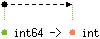Convert the given 64-bit integer (type `int64`) to an integer (type `int`). On 64-bit platforms, the 64-bit integer is taken modulo 263, i.e. the high-order bit is lost during the conversion. On 32-bit platforms, the 64-bit integer is taken modulo 231, i.e. the top 33 bits are lost during the conversion.

val of_float : 'a float -> 'a int64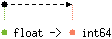Convert the given floating-point number to a 64-bit integer, discarding the fractional part (truncate towards 0). The result of the conversion is undefined if, after truncation, the number is outside the range [`Int64.min_int`, `Int64.max_int`].

val to_float : 'a int64 -> 'a float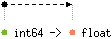Convert the given 64-bit integer to a floating-point number.

val of_int32 : 'a int32 -> 'a int64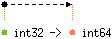Convert the given 32-bit integer (type `int32`) to a 64-bit integer (type `int64`).

val to_int32 : 'a int64 -> 'a int32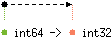Convert the given 64-bit integer (type `int64`) to a 32-bit integer (type `int32`). The 64-bit integer is taken modulo 232, i.e. the top 32 bits are lost during the conversion.

val of_nativeint : 'a nativeint -> 'a int64Convert the given native integer (type `nativeint`) to a 64-bit integer (type `int64`).

val to_nativeint : 'a int64 -> 'a nativeint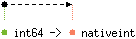Convert the given 64-bit integer (type `int64`) to a native integer. On 32-bit platforms, the 64-bit integer is taken modulo 232. On 64-bit platforms, the conversion is exact.

val of_string : 'a string -{'b | Failure: 'b |}-> 'a int64
with 'a < 'b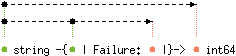Convert the given string to a 64-bit integer. The string is read in decimal (by default) or in hexadecimal, octal or binary if the string begins with `0x`, `0o` or `0b` respectively. Raise `Failure "int_of_string"` if the given string is not a valid representation of an integer.

val to_string : 'a int64 -> 'a string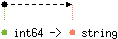Return the string representation of its argument, in decimal.

val bits_of_float : 'a float -> 'a int64Return the internal representation of the given float according to the IEEE 754 floating-point ``double format'' bit layout. Bit 63 of the result represents the sign of the float; bits 62 to 52 represent the (biased) exponent; bits 51 to 0 represent the mantissa.

val float_of_bits : 'a int64 -> 'a floatReturn the floating-point number whose internal representation, according to the IEEE 754 floating-point ``double format'' bit layout, is the given `int64`.

type (#'a:level) t = 'a int64
An alias for the type of 64-bit integers.

val compare : 'a t -> 'a t -> 'a int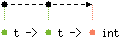The comparison function for 64-bit integers, with the same specification as `Pervasives.compare`. Along with the type `t`, this function `compare` allows the module `Int64` to be passed as argument to the functors `Set.Make` and `Map.Make`.